# B-spline Basis Functions: Important PropertiesLet us recall the definition of the B-spline basis functions as follows: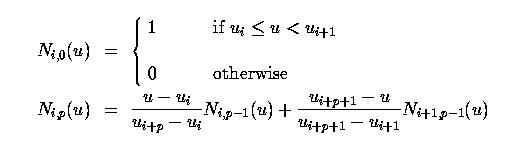This set of basis functions has the following properties, many of which resemble those of Bézier basis functions.

1. Ni,p(u) is a degree p polynomial in u
2. Nonnegativity -- For all i, p and u, Ni,p(u) is non-negative
3. Local Support -- Ni,p(u) is a non-zero polynomial on [ui,ui+p+1)
This has been discussed on previous page.
4. On any span [ui, ui+1), at most p+1 degree p basis functions are non-zero, namely: Ni-p,p(u), Ni-p+1,p(u), Ni-p+2,p(u), ..., and Ni,p(u)
5. Partition of Unity -- The sum of all non-zero degree p basis functions on span [ui, ui+1) is 1:
The previous property shows that Ni-p,p(u), Ni-p+1,p(u), Ni-p+2,p(u), ..., and Ni,p(u) are non-zero on [ui, ui+1). This one states that the sum of these p+1 basis functions is 1.
6. If the number of knots is m+1, the degree of the basis functions is p, and the number of degree p basis functions is n+1, then m = n + p + 1 :
This is not difficult to see. Let Nn,p(u) be the last degree p basis function. It is non-zero on [un, un+p+1). Since it is the last basis function, un+p+1 must be the last knot um. Therefore, we have un+p+1 = um and n + p + 1 = m. In summary, given m and p, let n = m - p - 1 and the degree p basis functions are N0,p(u), N1,p(u), N2,p(u), ..., and Nn,p(u).
7. Basis function Ni,p(u) is a composite curve of degree p polynomials with joining points at knots in [ui, ui+p+1 )
The example shown on the previous page illustrates this property well. For example, N0,2(u), which is non-zero on [0,3), is constructed from three parabolas defined on [0,1), [1,2) and [2,3). They are connected together at the knots 2 and 3.
8. At a knot of multiplicity k, basis function Ni,p(u) is Cp-k continuous.
Therefore, increasing multiplicity decreases the level of continuity, and increasing degree increases continuity. The above mentioned degree two basis function N0,2(u) is C1 continuous at knots 2 and 3, since they are simple knots (k = 1).### The Impact of Multiple Knots

Multiple knots do have significant impact on the computation of basis functions and some "counting" properties. We shall look at two of them and a calculation example will be provided on the next page.
1. Each knot of multiplicity k reduces at most k-1 basis functions' non-zero domain.
Consider Ni,p(u) and Ni+1,p(u). The former is non-zero on [ui, ui+p+1) while the latter is non-zero on [ui+1, ui+p+2). If we move ui+p+2 to ui+p+1 so that they become a double knot. Then, Ni,p(u) still has p+1 knot spans on which it is non-zero; but, the number of knot spans on which Ni+1,p(u) is non-zero is reduced by one because the span [ui+p+1,ui+p+2) disappears.

This observation can be generalized easily. In fact, ignoring the change of knot span endpoints, to create a knot of multiplicity k, k-1 basis functions will be affected. One of them loses one knot span, a second of them loses two, a third of them loses three and so on.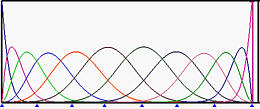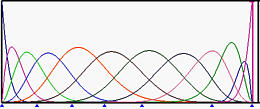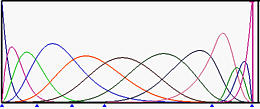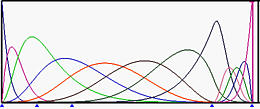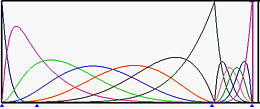The above shows the basis functions of degree 5 where the left end and right end knots have multiplicity 6, while all middle knots are simple (left on top row). The top right figure is the result of moving u5 to u6. Those basis functions ended at u6 have fewer knot spans on which they are non-zero. In the middle row figures, u4 and then u3 are moved to u6, making u6 a knot of multiplicity 4. The bottom row figure shows the result after moving u2 to u6, creating a knot of multiplicity 5.

2. At each internal knot of multiplicity k, the number of non-zero basis functions is at most p - k + 1, where p is the degree of the basis functions.
Since moving ui-1 to ui will pull a basis function whose non-zero ends at ui-1 to end at ui, this reduces the number of non-zero basis function at ui by one. More precisely, increasing ui's multiplicity by one will decrease the number of non-zero basis functions by one. Since there are at most p+1 basis functions can be non-zero at ui, the number of non-zero basis functions at a knot of multiplicity k is at most (p + 1) - k = p - k + 1.

In the above figures, since the multiplicity of knot u6 are 1 (simple), 2, 3, 4 and 5, the numbers of non-zero basis function at u6 are 5, 4, 3, 2 and 1.